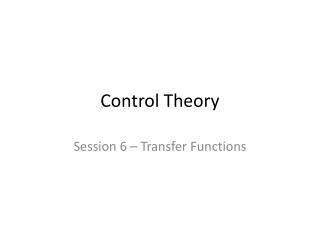DownloadDownload PresentationControl Theory

# Control Theory

Télécharger la présentation## Control Theory

- - - - - - - - - - - - - - - - - - - - - - - - - - - E N D - - - - - - - - - - - - - - - - - - - - - - - - - - -
##### Presentation Transcript

1. ControlTheory Session6 – Transfer Functions

2. Standard form of first order TF We willconsider changes with respect to a giveninitial equilibrium Step response:

3. Step response of Δz(t) A t B Definition of step response: Δz(t) ifΔu(t) is a step of size 1

4. Second order processes Typicalexample: mass-spring-damper z(t) u(t) (set-up in a horizontal plane, spring in rest positionwhen x=0, initialvelocity v=0: equilibrium!)

5. Standard form of second order TF

6. Step respones of 2nd order processes >1: Overdamped =1: Critically damped = fastest without oscillations <1: Underdamped: Oscillations! +

7. Overshoot in 2nd order systems

8. Overshoot in 2nd order systems

9. Group Task m=1 [kg] k=1 [N/m] Find the TF and plot the step response for c= 4 [Ns/m] c=2 [Ns/m] c=1 [Ns/m]

10. Group Task 2 m=1 [kg] k=1 [N/m] Can we nowadd a P controller and calculate the transfer function of the closed loop? (by the way, what’s the transfer function of a P controller?)